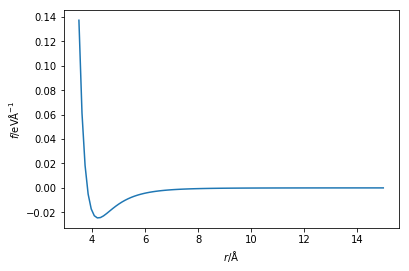## Cut-offs

Plotting the Lennard-Jones potential across a very large distance will give something similar to that shown below.

%matplotlib inline
import numpy as np
import matplotlib.pyplot as plt

def lj_force(r, epsilon, sigma):
"""
Implementation of the Lennard-Jones potential
to calculate the force of the interaction.

Parameters
----------
r: float
Distance between two particles (Å)
epsilon: float
Potential energy at the equilibrium bond
length (eV)
sigma: float
Distance at which the potential energy is
zero (Å)

Returns
-------
float
Force of the van der Waals interaction (eV/Å)
"""
return 48 * epsilon * np.power(
sigma / r, 13) - 24 * epsilon * np.power(
sigma / r, 7)

r = np.linspace(3.5, 15, 100)
plt.plot(r, lj_force(r, 0.0103, 3.4))
plt.xlabel(r'$r$/Å')
plt.ylabel(r'$f$/eVÅ$^{-1}$')
plt.show()It is clear that as the particles move further away from each other, the force between them tends towards zero. The concept of a cut-off suggests that if two particles are found to be very far apart, there is no need to calculate thh energy/force. Instead the value is simply taken as 0,

When there are periodic boundaries present (mentioned later), the cut-off ensures that the forces are not sampled incorrectly by having a cut-off of less than half of the cell size.

This Python code below shows a modification of the Lennard-Jones potential that accounts for this cut-off.

cutoff = 15

def lj_force_cutoff(r, epsilon, sigma):
"""
Implementation of the Lennard-Jones potential
to calculate the force of the interaction which
is considerate of the cut-off.

Parameters
----------
r: float
Distance between two particles (Å)
epsilon: float
Potential energy at the equilibrium bond
length (eV)
sigma: float
Distance at which the potential energy is
zero (Å)

Returns
-------
float
Force of the van der Waals interaction (eV/Å)
"""
if r < cutoff:
return 48 * epsilon * np.power(
sigma / r, 13) - 24 * epsilon * np.power(
sigma / r, 7)
else:
return 0